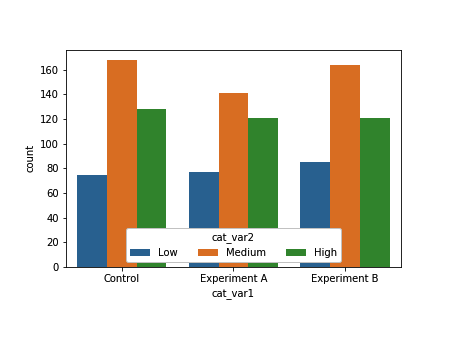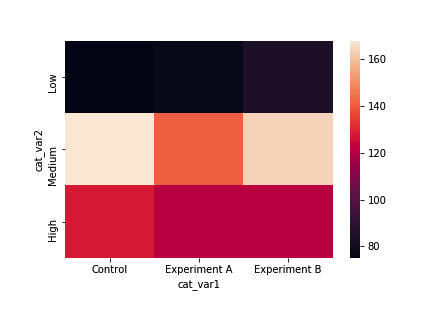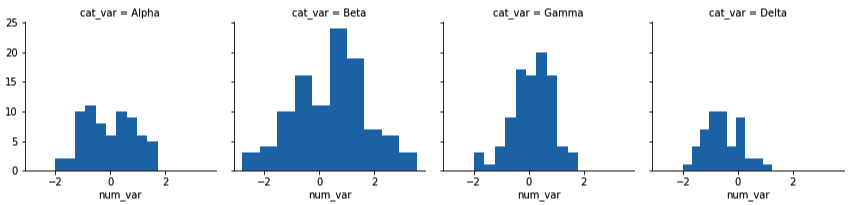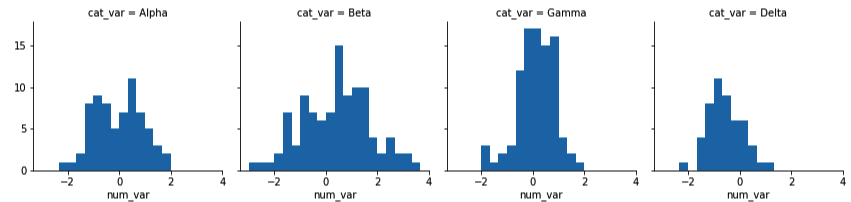Python：matplotlib 和 Seaborn 之簇状柱形图、分面 (三十五)

簇状柱形图

sb.countplot(data = df, x = 'cat_var1', hue = 'cat_var2')ax = sb.countplot(data = df, x = 'cat_var1', hue = 'cat_var2')
ax.legend(loc = 8, ncol = 3, framealpha = 1, title = 'cat_var2')其他方法（热图）

ct_counts = df.groupby(['cat_var1', 'cat_var2']).size()
ct_counts = ct_counts.reset_index(name = 'count')
ct_counts = ct_counts.pivot(index = 'cat_var2', columns = 'cat_var1', values = 'count')
sb.heatmap(ct_counts)sb.heatmap(ct_counts, annot = True, fmt = 'd')

annot = True 可以在每个单元格中显示注释，但是默认的字符串格式精度只能达到小数点后两位。添加 fmt = 'd' 表示注释将全变成整数形式。

分面

g = sb.FacetGrid(data = df, col = 'cat_var')
g.map(plt.hist, "num_var")bin_edges = np.arange(-3, df['num_var'].max()+1/3, 1/3)
g = sb.FacetGrid(data = df, col = 'cat_var')
g.map(plt.hist, "num_var", bins = bin_edges)